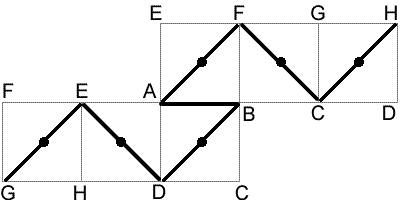#### You may also like### All in the Mind

Imagine you are suspending a cube from one vertex and allowing it to hang freely. What shape does the surface of the water make around the cube?### Just Rolling Round

P is a point on the circumference of a circle radius r which rolls, without slipping, inside a circle of radius 2r. What is the locus of P?### Coke Machine

The coke machine in college takes 50 pence pieces. It also takes a certain foreign coin of traditional design...

# Hamiltonian Cube

##### Age 11 to 16 ShortChallenge LevelThe image above shows a possible path. Each edge joining a corner to a face centre has length $\frac{1}{\sqrt{2}}$ (by Pythagoras' Theorem), while each edge which joins two adjacent corners has length $1$. So the length of the path above is $1+6\sqrt{2}$. This is the length of the shortest path to pass through all the vertices.

To prove this, note the length of the shortest path must be at least $\frac{13}{\sqrt{2}}$. Such a path would move alternately between corners and faces, but as there are $8$ corners and only $6$ faces, so this is impossible. So at least one of the edges must join to corners, and so the shortest length is $1+6\sqrt{2}$.
This problem is taken from the UKMT Mathematical Challenges.
You can find more short problems, arranged by curriculum topic, in our short problems collection.